# Simple harmonic motion Questions and Answers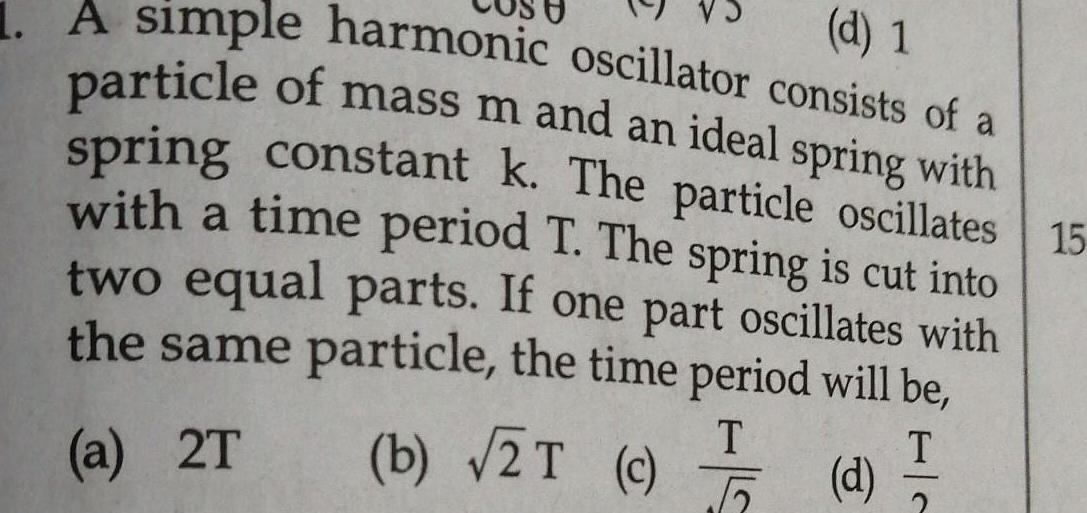Physics
Simple harmonic motion
d 1 1 A simple harmonic oscillator consists of a particle of mass m and an ideal spring with spring constant k The particle oscillates 15 with a time period T The spring is cut into two equal parts If one part oscillates with the same particle the time period will be T T a 2T b 2T c 5 d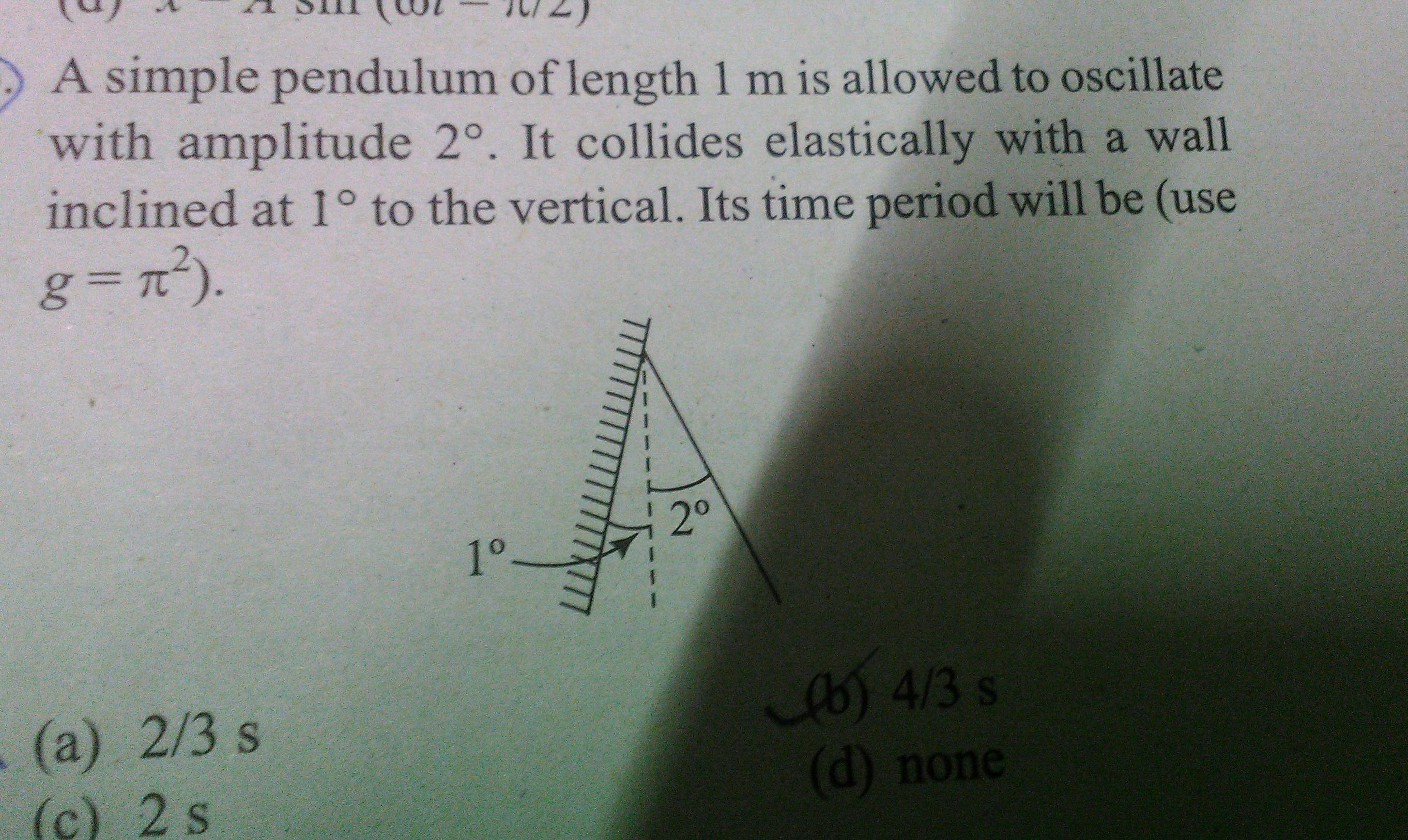Physics
Simple harmonic motion
A simple pendulum of length 1 m is allowed to oscillate with amplitude 2 It collides elastically with a wall inclined at 1 to the vertical Its time period will be use g n a 2 3 s c 2 s 1 2 b 4 3 s d none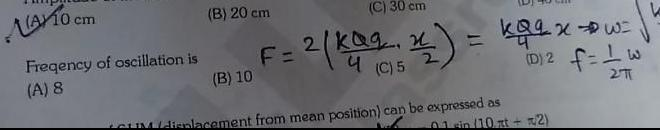Physics
Simple harmonic motion
A10 cm Freqency of oscillation is A 8 B 20 cm B 10 C 30 cm F 2 kaa 2 C 5 kaq xw D 2 f 1 w 271 01 sin 10xt 2 CUM displacement from mean position can be expressed as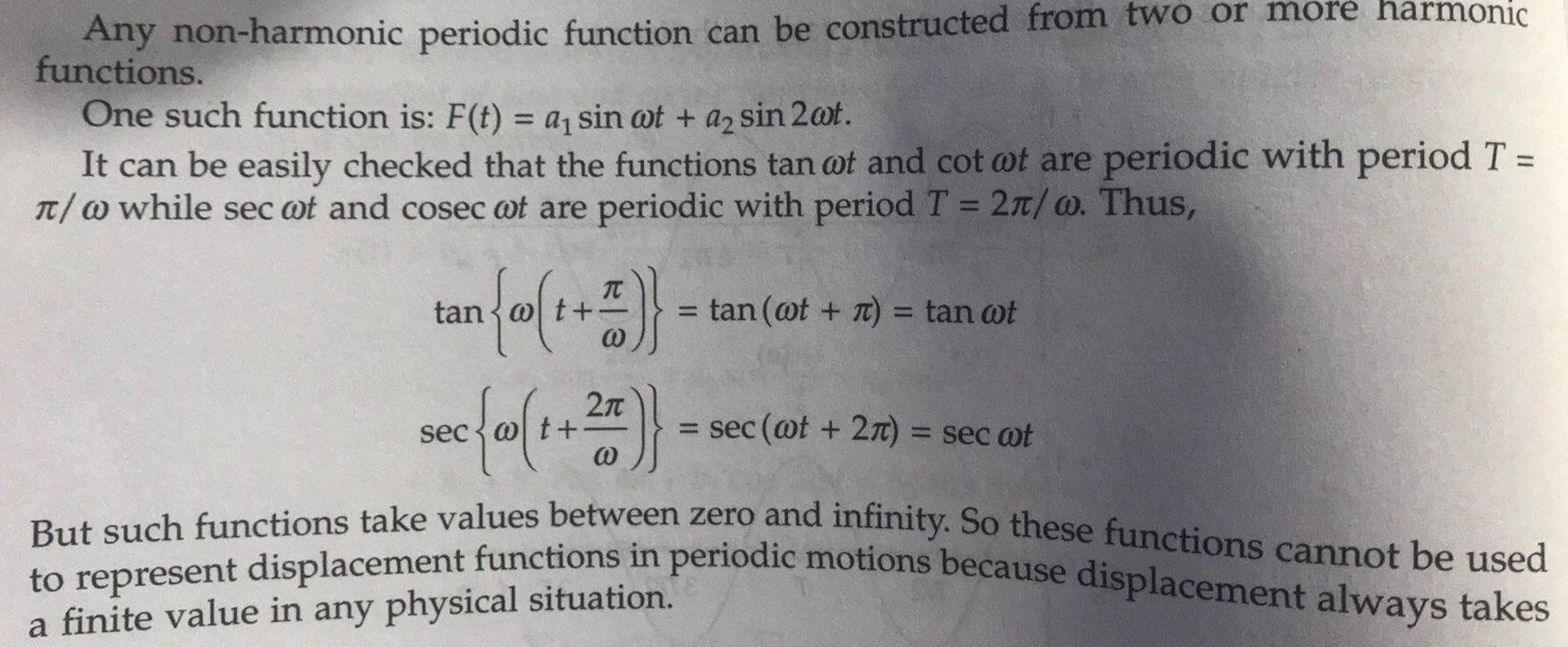Physics
Simple harmonic motion
Any non harmonic periodic function can be constructed from two or more harmonic functions One such function is F t a sin at a sin 2 t It can be easily checked that the functions tan wot and cot at are periodic with period T w while sec wot and cosec wt are periodic with period T 2 w Thus an sec 1 2 But such functions take values between zero and infinity So these functions cannot be used to represent displacement functions in periodic motions because displacement always takes a finite value in any physical situation 1 tan wt 7 tan ot sec wt 2 sec wt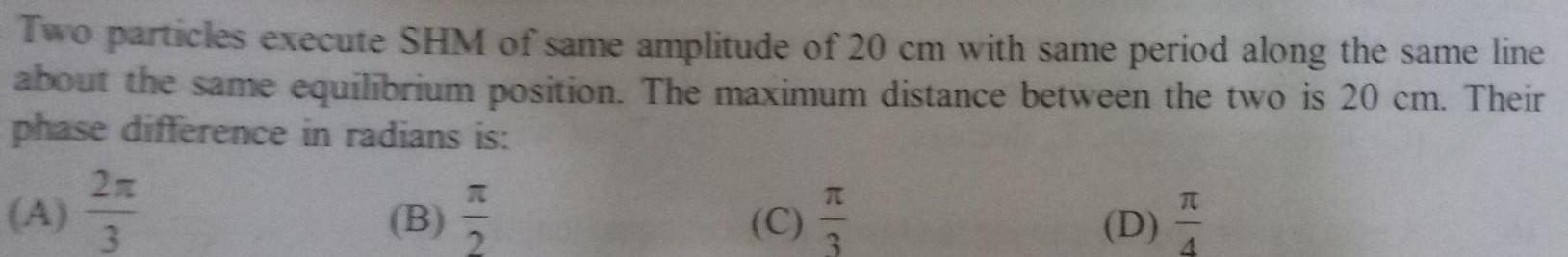Physics
Simple harmonic motion
Two particles execute SHM of same amplitude of 20 cm with same period along the same line about the same equilibrium position The maximum distance between the two is 20 cm Their phase difference in radians is 373 3 B N C D RT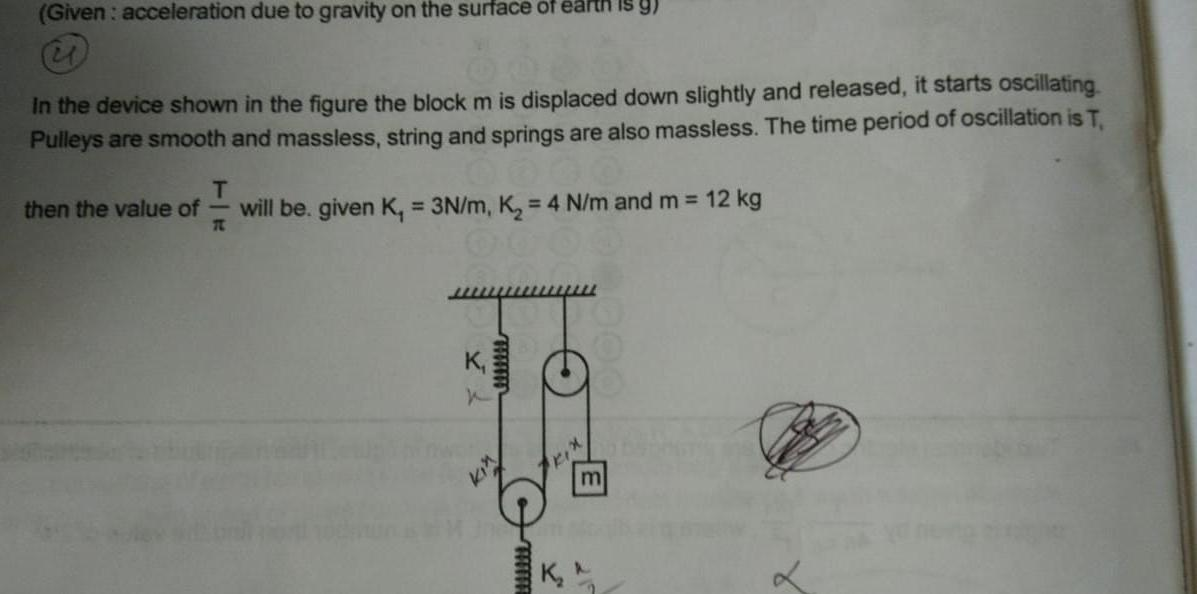Physics
Simple harmonic motion
Given acceleration due to gravity on the surface of earth is y i In the device shown in the figure the block m is displaced down slightly and released it starts oscillating Pulleys are smooth and massless string and springs are also massless The time period of oscillation is T T then the value of will be given K 3N m K 4 N m and m 12 kg T 00 Y wwwww K www Kix m K M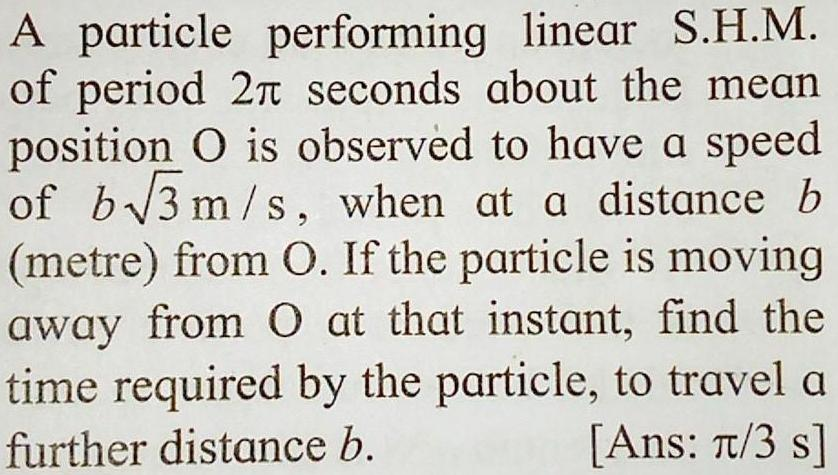Physics
Simple harmonic motion
A particle performing linear S H M of period 27 seconds about the mean position O is observed to have a speed of b 3 m s when at a distance b metre from O If the particle is moving away from O at that instant find the time required by the particle to travel a further distance b Ans T 3 s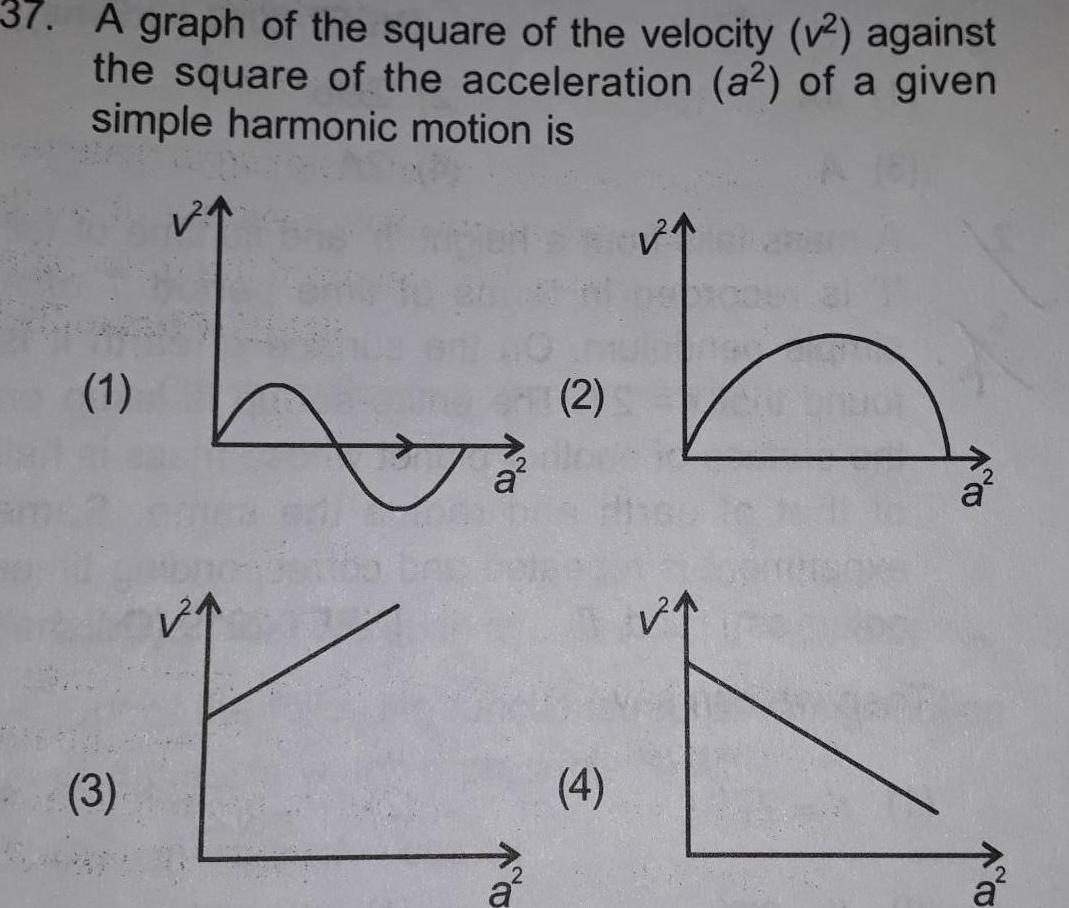Physics
Simple harmonic motion
37 A graph of the square of the velocity v against the square of the acceleration a of a given simple harmonic motion is 1 3 h h 2 a a 4 a CO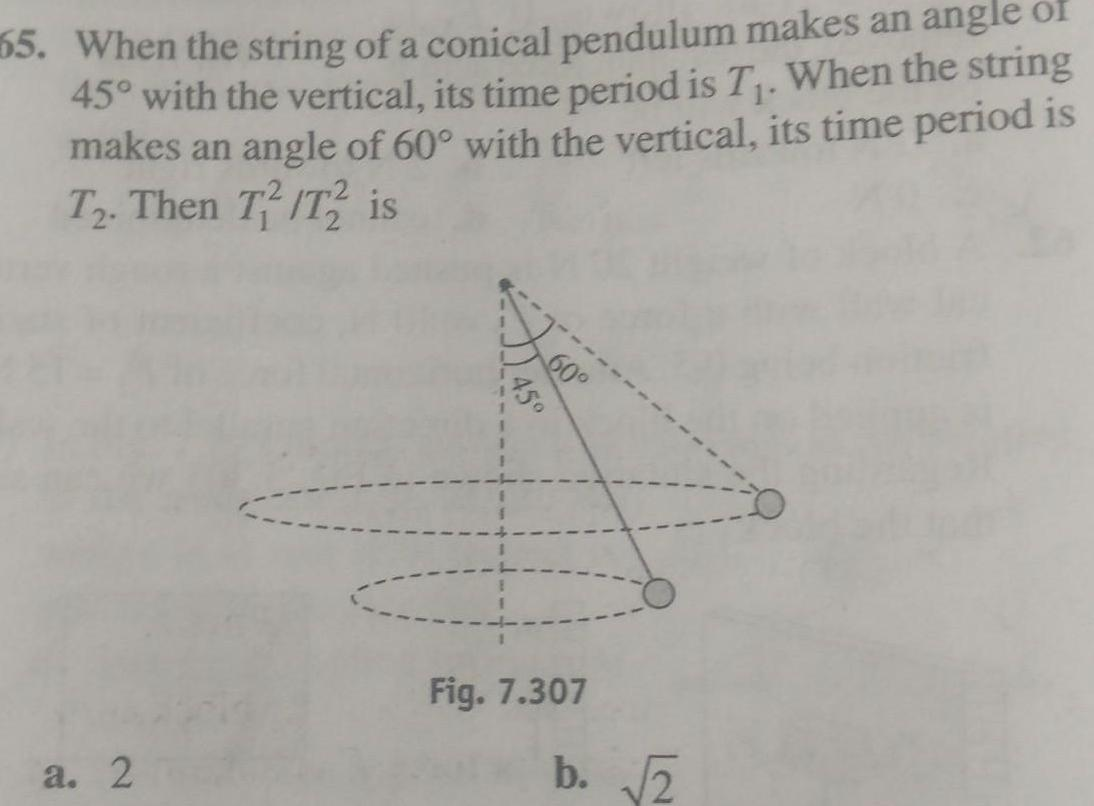Physics
Simple harmonic motion
55 When the string of a conical pendulum makes an angle of 45 with the vertical its time period is T When the string makes an angle of 60 with the vertical its time period is T2 Then T T2 is a 2 60 Fig 7 307 b 2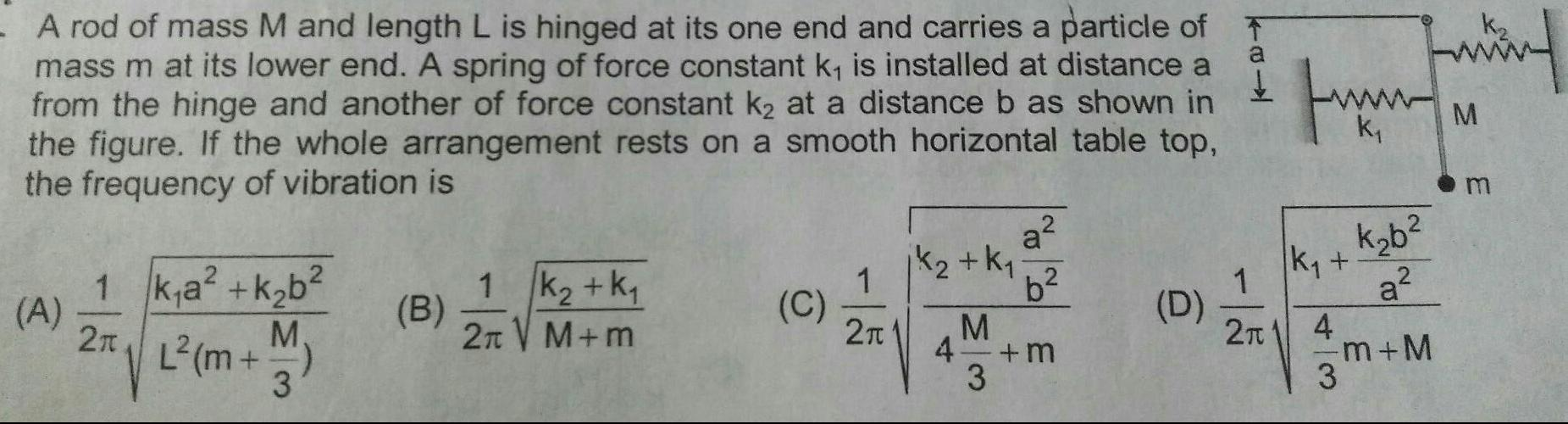Physics
Simple harmonic motion
A rod of mass M and length L is hinged at its one end and carries a particle of mass m at its lower end A spring of force constant k is installed at distance a from the hinge and another of force constant k at a distance b as shown in the figure If the whole arrangement rests on a smooth horizontal table top the frequency of vibration is A 1 k a k b 15 2T L m M 3 B 1 K k 2 V M m C jk K 1 2 M 4 3 a b 3 6 fum k K 1 21 4 3 k b a m M m nd M m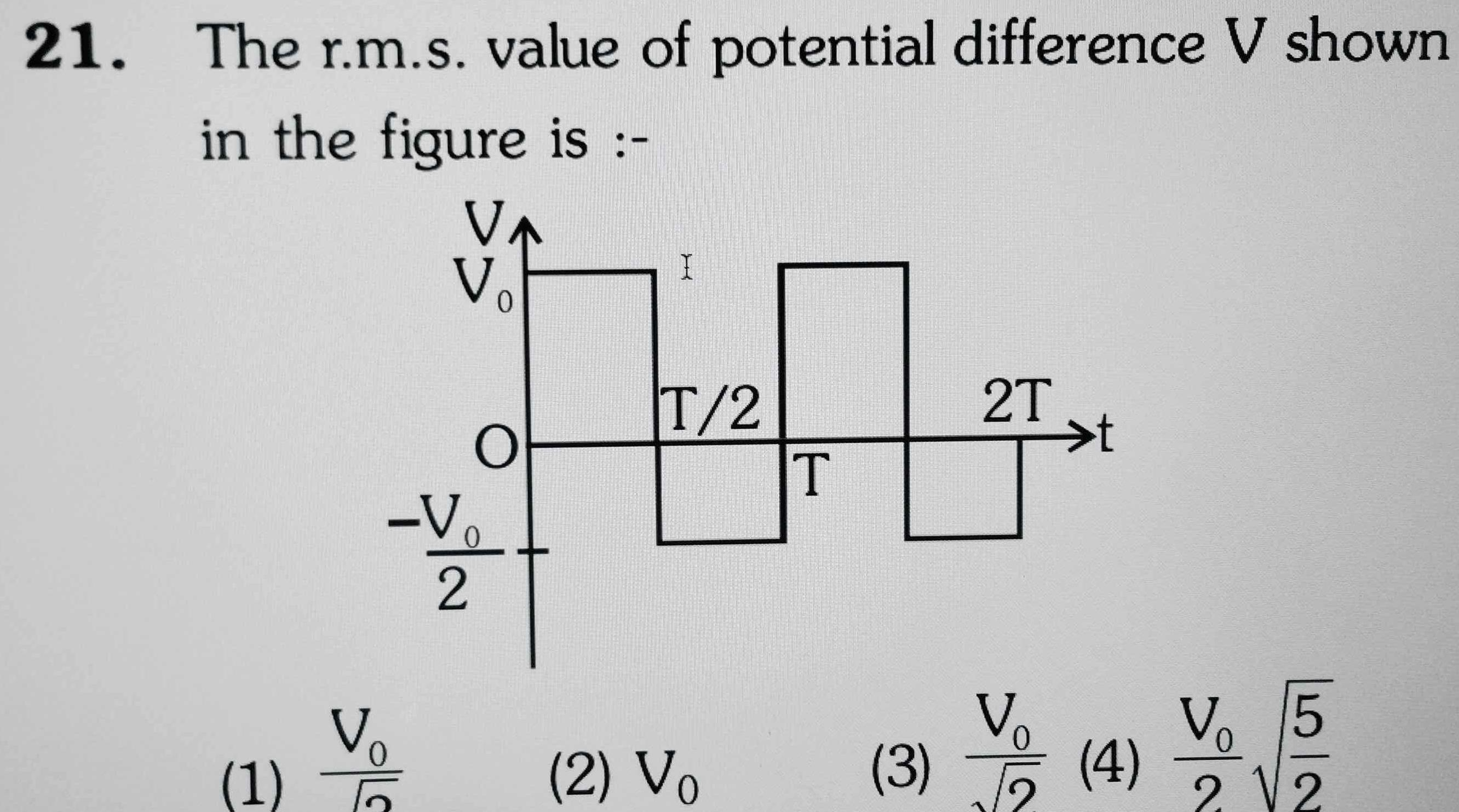Physics
Simple harmonic motion
21 The r m s value of potential difference V shown in the figure is 1 V Dole Vo 0 V 2 I T 2 2 Vo T 2T t 3 4 V 5 2 2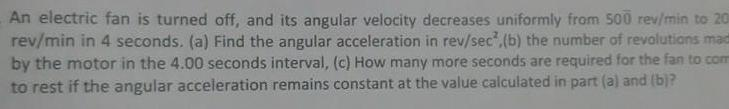Physics
Simple harmonic motion
An electric fan is turned off and its angular velocity decreases uniformly from 500 rev min to 20 rev min in 4 seconds a Find the angular acceleration in rev sec b the number of revolutions mad by the motor in the 4 00 seconds interval c How many more seconds are required for the fan to com to rest if the angular acceleration remains constant at the value calculated in part a and b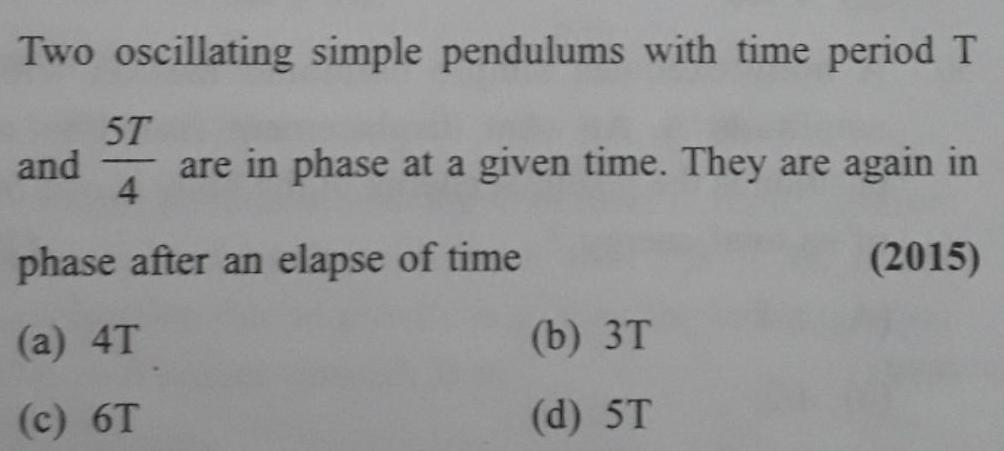Physics
Simple harmonic motion
Two oscillating simple pendulums with time period T 5T and are in phase at a given time They are again in 4 phase after an elapse of time a 4T c 6T b 3T d 5T 2015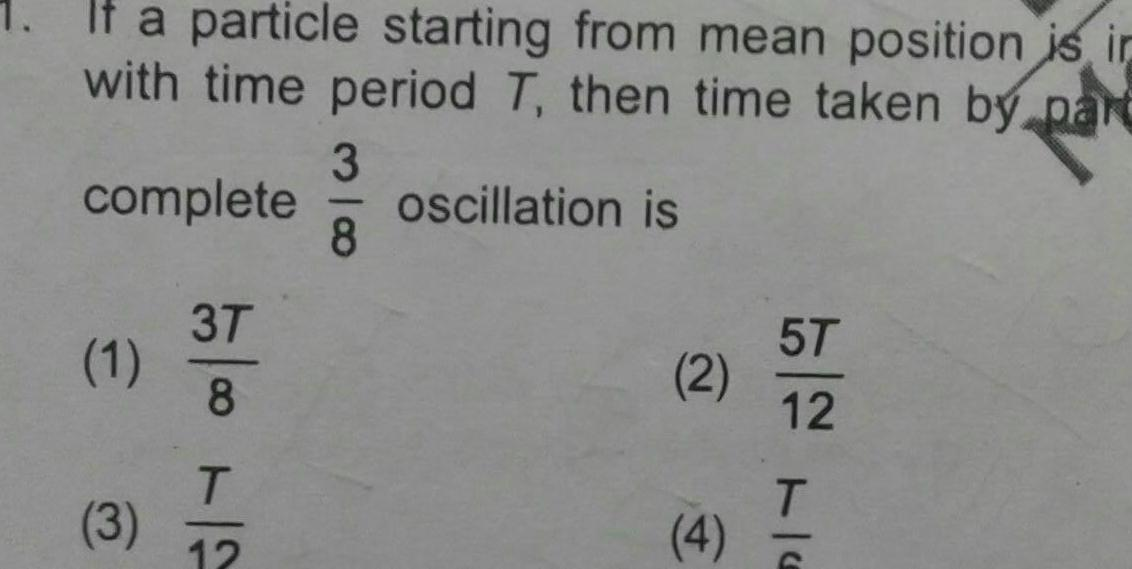Physics
Simple harmonic motion
If a particle starting from mean position is in with time period T then time taken by part oscillation is complete 1 3 3T 8 T 12 3 8 2 4 5T 12 TPhysics
Simple harmonic motion
If two SHMs are represented by equations TC 5 3 Y 4 sin 3nt Y 4 sin 3rt 3 cos 3nt then the ratio of their amplitudes is 1 1 2 2 1 1 3 3 1 4 1 3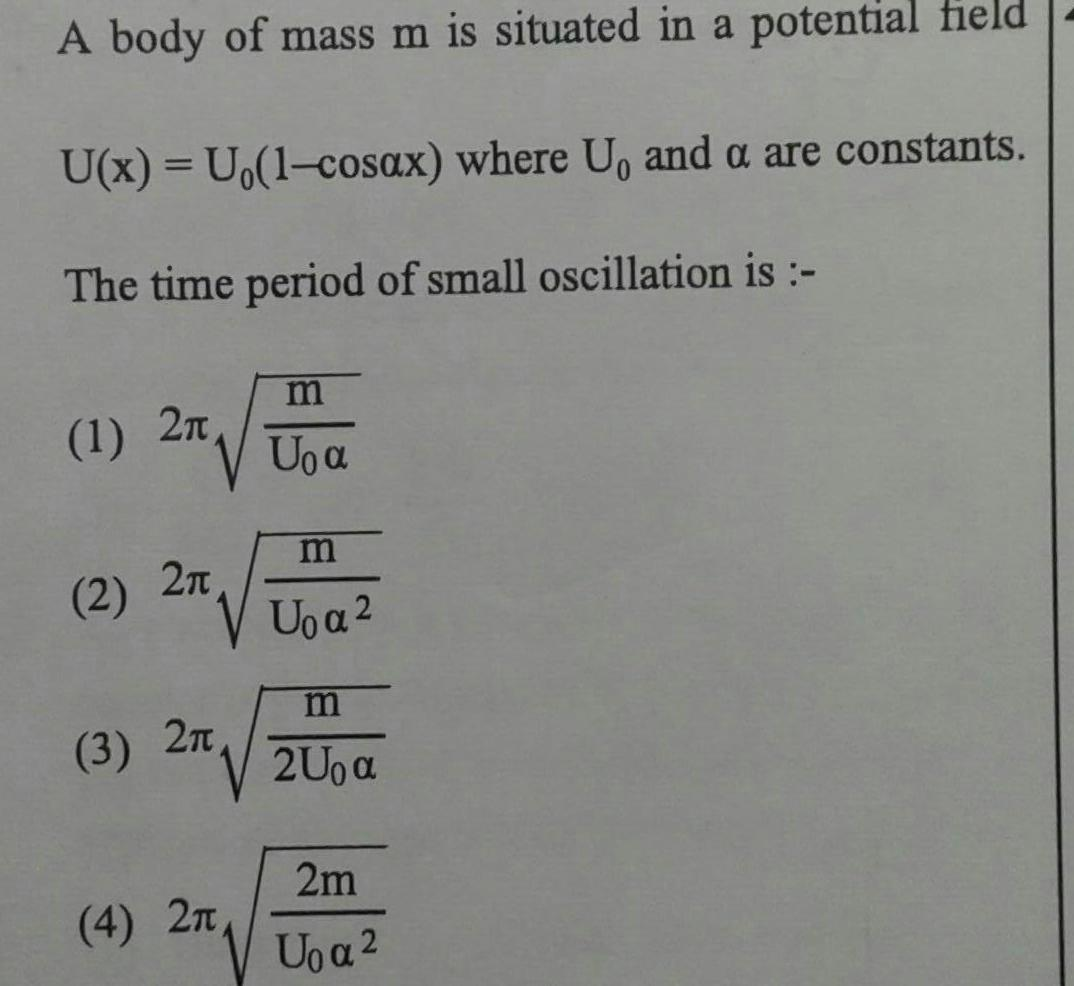Physics
Simple harmonic motion
A body of mass m is situated in a potential field U x U 1 cosax where U and a are constants The time period of small oscillation is m 1 2 Uoa 2 2n 3 2T 4 2 m U a m 2U a 2m U a